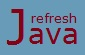# Operator Precedence in Java with Example

In real life when we solve an expression containing multiple operators we follow some rules. For example in an expression `2+3*4`, we do the multiplication first before addition because the multiplication operator has higher precedence than addition operator. The same applies in java as well.

In java, operator precedence is a rule that tells us the precedence of different operators. Operators with higher precedence are evaluated before operators with lower precedence. For example in expression `a+b*c`, the operator `*` will be evaluated before `+` operator, because operator `*` has higher precedence than `+` operator.

Table bellow shows the precedence of operators in decreasing order, the operators appearing first in table have higher precedence than operators appearing later. Operators appearing in same row of table have equal precedence. When operators of equal precedence appears in the same expression, a rule governs the evaluation order which says that all binary operators except for the assignment operator are evaluated from left to right while assignment operator is evaluated from right to left. For example in expression `2+3-4`, 2 and 3 is added first then 4 is subtracted from it. Consider `a` and `b` as two operands for the examples given in below table.

## Java operator precedence table

Operators Precedence Example
Postfix `expr++ expr--` a++ , a--
Unary `++expr --expr +expr -expr ~ !` ++a , --a , !a
Multiplicative `* / %` a*b , a/b , a%b
Additive `+ -` a+b , a-b
Shift `<< >> >>>` a<<2 , a>>1
Relational `< > <= >= instanceof` a<2 , a>1
Equality `== !=` a==b , a!=b
Bitwise AND `&` a&b
Bitwise exclusive OR `^` a^b
Bitwise inclusive OR `|` a|b
Logical AND `&&` a&&b
Logical OR `||` a||b
Ternary `? :` a = a>2 ? a : b
Assignment `= += -= *= /= %= &= ^= |= <<= >>= >>>=` a=b, a+=b, a/=b, a>>=2

### Which operator has highest precedence in java ?

The postfix operator(eg. a++, a--) has the highest precedence in java.

### Which operator has lowest precedence in java ?

Assignment operator and it's different forms has the lowest precedence in java.

A programmer should remember bellow rules while evaluating an expression in java.

• The operands of operators are evaluated from left to right. For example in expression `++a + b--`, the operand `++a` is evaluated first then `b--` is evaluated.
• Every operand of an operator (except the conditional operators `&&`, `||`, and `? :`) are evaluated completely before any part of the operation itself is performed. For example in expression `++a + b--`, the addition operation will take place only after `++a` and `b--` is evaluated.
• The left-hand operand of a binary operator are evaluated completely before any part of the right-hand operand is evaluated.
• Order of evaluation given by parenthesis `()` get's preference over operator precedence.
• The prefix version of `++` or `--` evaluates/uses the incremented value in an expression while postfix version of `++` or `--` evaluates the current value, then increases/decreases the operand value.
• All binary operators are evaluated from left to right except assignment operator.

### What changes the precedence of the operators in Java ?

Parentheses "()" are used to alter the order of evaluation. For example in an expression `a+b*c`, if you want the addition operation to take place first, then rewrite the expression as `(a+b)*c.`

### Java Program of Operator Precedence

```` class` OperatorPrecedence {
`public static void` main (String[] args) {
`int` result = 0;
result = 5 + 2 * 3 - 1;
System.out.println(`"5 + 2 * 3 - 1 = "` +result);

result = 5 + 4 / 2 + 6;
System.out.println(`"5 + 4 / 2 + 6 = "` +result);

result = 3 + 6 / 2 * 3 - 1 + 2;
System.out.println(`"3 + 6 / 2 * 3 - 1 + 2 = "` +result);

result = 6 / 2 * 3 * 2 / 3;
System.out.println(`"6 / 2 * 3 * 2 / 3 = "` +result);

`int` x = 2;
result = x++ + x++ * --x / x++ - --x + 3 >> 1 | 2;
System.out.println(`"result = "` +result);
}
}
```

Output:

5 + 2 * 3 - 1 = 10
5 + 4 / 2 + 6 = 13
3 + 6 / 2 * 3 - 1 + 2 = 13
6 / 2 * 3 * 2 / 3 = 6
result = 2

Let's understand how the last expression `x++ + x++ * --x / x++ - --x + 3 >> 1 | 2` is evaluated step by step.

````  operands are evaluated first, left to right`
2 + 3 * 3 / 3 - 3 + 3 >> 1 | 2
`  * and / have higher preference than other, * evaluated first as it comes in left`
2 + 9 / 3 - 3 + 3 >> 1 | 2
`  / have higher preference than others`
2 + 3 - 3 + 3 >> 1 | 2
`  + and - have higher preference than others`
5 >> 1 | 2
`  >> have higher preference than |`
2 | 2
`  Output`
2 ```
★★★
• Nested parentheses in an expression are evaluated from the innermost parentheses to the outermost parenthesis.
• If evaluation of the left-hand operand of a binary operator does not complete properly, no part of the right-hand operand will be evaluated.
• Arguments in a method are evaluated from left to right.